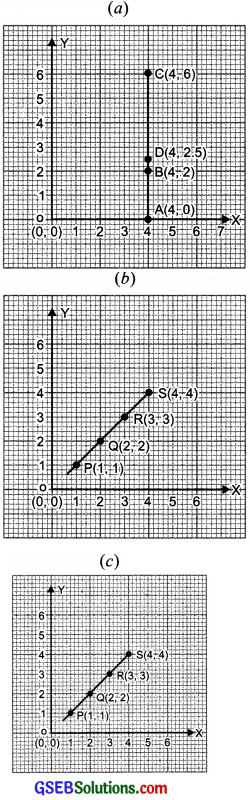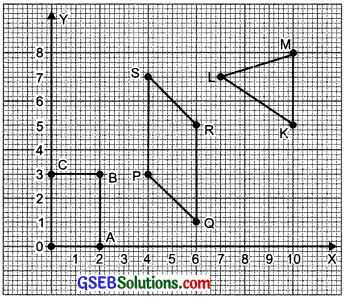# GSEB Solutions Class 8 Maths Chapter 15 Introduction to Graphs Ex 15.2

Gujarat Board GSEB Textbook Solutions Class 8 Maths Chapter 15 Introduction to Graphs Ex 15.2 Questions and Answers.

## Gujarat Board Textbook Solutions Class 8 Maths Chapter 15 Introduction to Graphs Ex 15.2

Question 1.
Plot the following points on a graph sheet. Verify if they lie on a line:
(a) A (4, 0), B(4, 2), C(4, 6), D(4, 2.5)
(b) P(1, 1), Q(2, 2), R(3, 3), S(4, 4)
(c) K(2, 3), L(5, 3), M(5, 5), N(2, 5)
Solution:
In each case we draw the x-axis and the y-axis and plot the given point.
(a) On plotting the points A(4, 0), 5(4, 2), C(4, 6) and D(4, 2.5) and then joining them we find that they all lie on the same line.
(b) On plotting the points P(1, 1), Q(2, 2), 5(3, 3) and 5(4, 4) and then joining them we find that they all lie on the same line.
(c) plotting the points K(2, 3) L(5, 3), M(5, 5) and N(2, 5) and joining them we find that all of them do not lie on the same line.Question 2.
Draw the line passing through (2, 3) and (3, 2). Find the coordinates of the points at which this line meets the x-axis and y-axis?Solution:
On plotting the points A(2, 3) and B(3, 2) we draw a line that joins them. On extending, the line meets the x-axis at C(5, 0) and the y-axis at D(0, 5).

Question 3.
Write the coordinates of the vertices of each of these adjoining figures?Solution:
1. The coordinates of:
O are (0, 0) A are (2, 0)
B are (2, 3) C are (0, 3)

2. The coordinates of:
P are (4, 3) Q are (6, 1)
R are (6, 5) S are (4, 7)

3. The coordinates of:
K are (10, 5) L are (7, 7)
M are (10, 8)Question 4.
State whether true or false. Correct that are false?

1. A point whose x coordinate is zero and y-coordinate is non-zero will lie on the y-axis.
2. A point whose y coordinate is zero and x-coordinate is 5 will lie on y-axis.
3. The coordinates of the origin are (0, 0).

Solution:

1. True
2. False
3. True